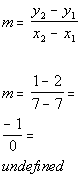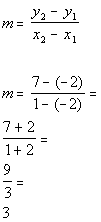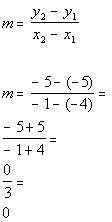TitleCollege Algebra
Answer/Discussion to Practice Problems
Tutorial 25: Slope of a LineAnswer/Discussion to 1a (2, -5) and (-1, -1)*Plug in x and y values into slope formula *Simplify

 Make sure that you are careful when one of your values is negative and you have to subtract it as we did in line 2.  -5 - (-1) is not the same as -5 - 1 and 2 - (-1) is not the same as 2 - 1.  The slope of the line is -4/3. Since the slope is negative, the line would fall (left to right).Answer/Discussion to 1b (7, 1) and (7, 2)*Plug in x and y values into slope formula *Simplify

 The slope of the line is undefined. Since the slope is undefined, the line is vertical.Answer/Discussion to 1c (1, 7) and (-2, -2)*Plug in x and y values into slope formula *Simplify

 Make sure that you are careful when one of your values is negative and you have to subtract it as we did in line 2.  7 - (-2) is not the same as 7 - 2 and 1 - (-2) is not the same as 1 - 2.  The slope of the line is 3. Since the slope is positive, the line would rise (left to right).Answer/Discussion to 1d (-1, -5) and (-4, -5)*Plug in x and y values into slope formula *Simplify

 Make sure that you are careful when one of your values is negative and you have to subtract it as we did in line 2.  -5 - (-5) is not the same as -5 - 5 and -1 - (-4) is not the same as -1 - 4.  The slope of the line is 0. Since the slope is zero, the line is horizontal.

Last revised on Jan. 16, 2010 by Kim Seward.
All contents copyright (C) 2002 - 2010, WTAMU and Kim Seward. All rights reserved.